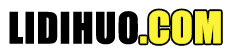# PL/SQL 函数

PL/SQL函数与PL/SQL过程非常相似。过程与函数之间的主要区别在于，函数必须始终返回值，另一方面，过程可能会也可能不会返回值。除此之外，PL/SQL过程的所有其他内容也适用于PL/SQL函数。

```CREATE [OR REPLACE] FUNCTION function_name [parameters]
[(parameter_name [IN | OUT | IN OUT] type [, ...])]
return return_datatype
{IS | AS}
BEGIN
< function_body >
END [function_name];```

function_name: 指定功能的名称。 [OR REPLACE] 选项允许修改现有功能。 optional parameter list包含参数的名称，模式和类型。 IN 表示将从外部传递值，而OUT表示此参数将用于在过程外部返回值。

### 该函数必须包含一个return语句。

RETURN子句指定您要从该函数返回的数据类型。 Function_body包含可执行部分。 使用AS关键字代替IS关键字来创建独立功能。

## PL/SQL函数示例

```create or replace function adder(n1 in number, n2 in number)
return number
is
n3 number(8);
begin
n3 :=n1+n2;
return n3;
end;
/  ```

```DECLARE
n3 number(2);
BEGIN
n3 := adder(11,22);
dbms_output.put_line('Addition is: ' || n3);
END;
/  ```

```Addition is: 33
Statement processed.
0.05 seconds```

## 另一个PL/SQL函数示例

```DECLARE
a number;
b number;
c number;
FUNCTION findMax(x IN number, y IN number)
return number
IS
z number;
BEGIN
if x > y THEN
z:= x;
ELSE
Z:= y;
END IF;
return z;
END;
BEGIN
a:= 23;
b:= 45;
c := findMax(a, b);
dbms_output.put_line(' Maximum of (23,45): ' || c);
END;
/```

```Maximum of (23,45): 45
Statement processed.
0.02 seconds```

## 使用表的PL/SQL函数示例

 Id 名称 部门 薪金 1 alex 网络开发人员 35000 2 ricky 程序开发人员 45000 3 mohan 网页设计师 35000 4 dilshad 数据库管理 44000

```CREATE OR REPLACE FUNCTION totalCustomers
return number IS
total number(2) := 0;
BEGIN
SELECT count(*) into total
FROM customers;
return total;
END;
/```

`Function created. `

```DECLARE
c number(2);
BEGIN
c := totalCustomers();
dbms_output.put_line('Total no. of Customers: ' || c);
END;
/```

```Total no. of Customers: 4
PL/SQL procedure successfully completed.```

## 计算数字阶乘的示例

```DECLARE
num number;
factorial number;
FUNCTION fact(x number)
return number
IS
f number;
BEGIN
if x=0 THEN
f := 1;
ELSE
f := x * fact(x-1);
END IF;
return f;
END;
BEGIN
num:= 6;
factorial := fact(num);
dbms_output.put_line(' Factorial '|| num || ' is ' || factorial);
END;
/```

```Factorial 6 is 720
PL/SQL procedure successfully completed.```

## PL/SQL删除函数

`DROP FUNCTION function_name;`
"内容整理不易，点下广告也是鼓励～"

Copyright © 2020 立地货 All Rights Reserved.